# 12V Car Battery Solar Charger

Charge 12V car battery using solar power and the Infineon 600V CoolMos C7 Gold superjunction MOSFET series G7.

IntermediateFull instructions provided2 days673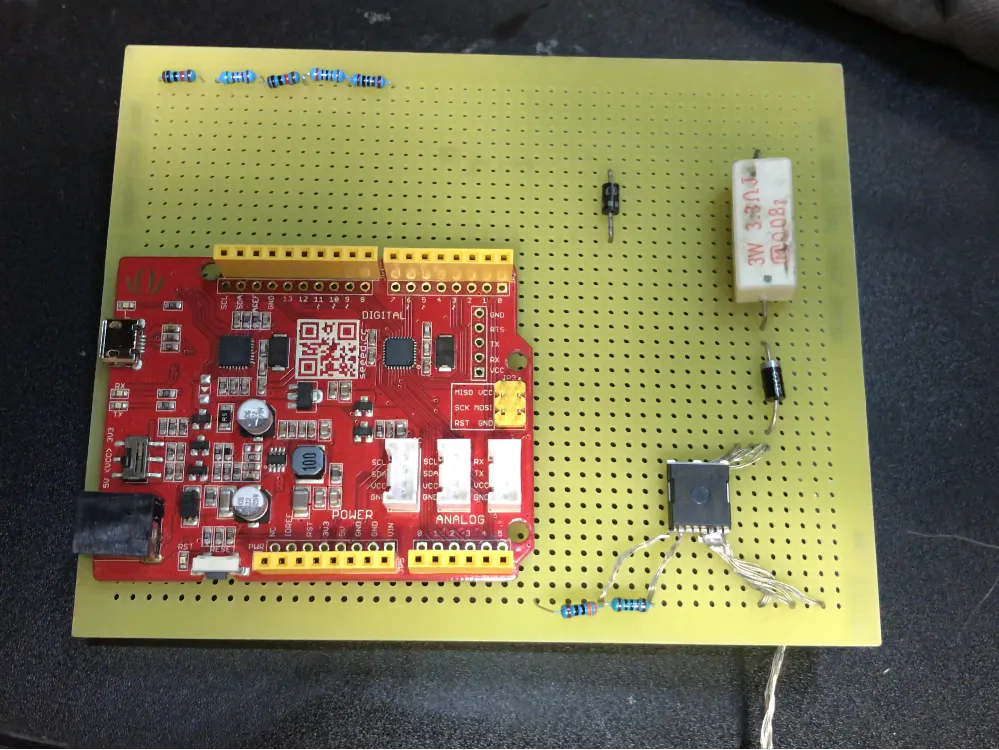## Things used in this project

### Hardware components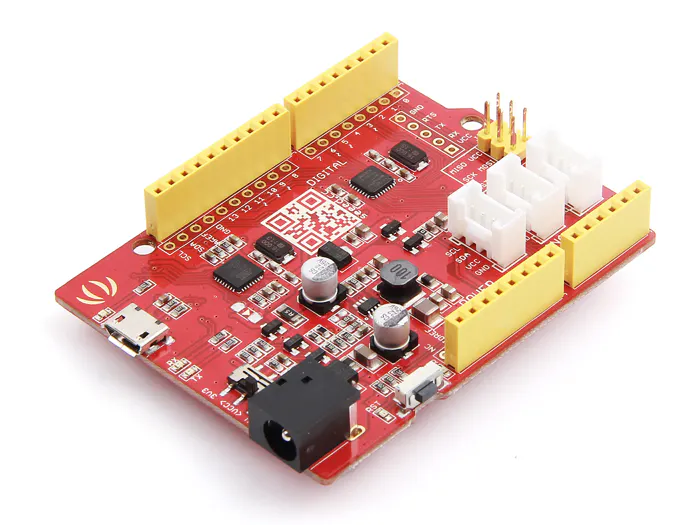Seeeduino V4.2
×1Resistor 1k ohm
×1
 Resistor 5.7k ohm
×1
 Resistor 2k ohm
×1Resistor 10k ohm
×1
 Diode B4304-03
×1
 Diode RL205
×1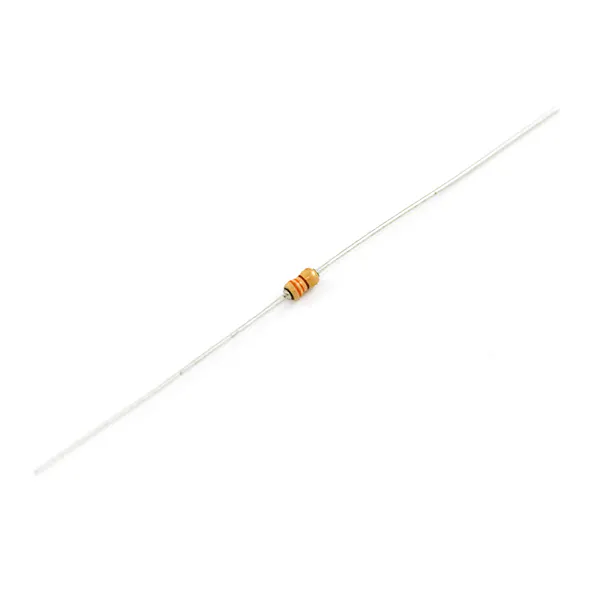Resistor 330 ohm
×1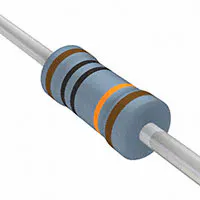Resistor 100k ohm
×1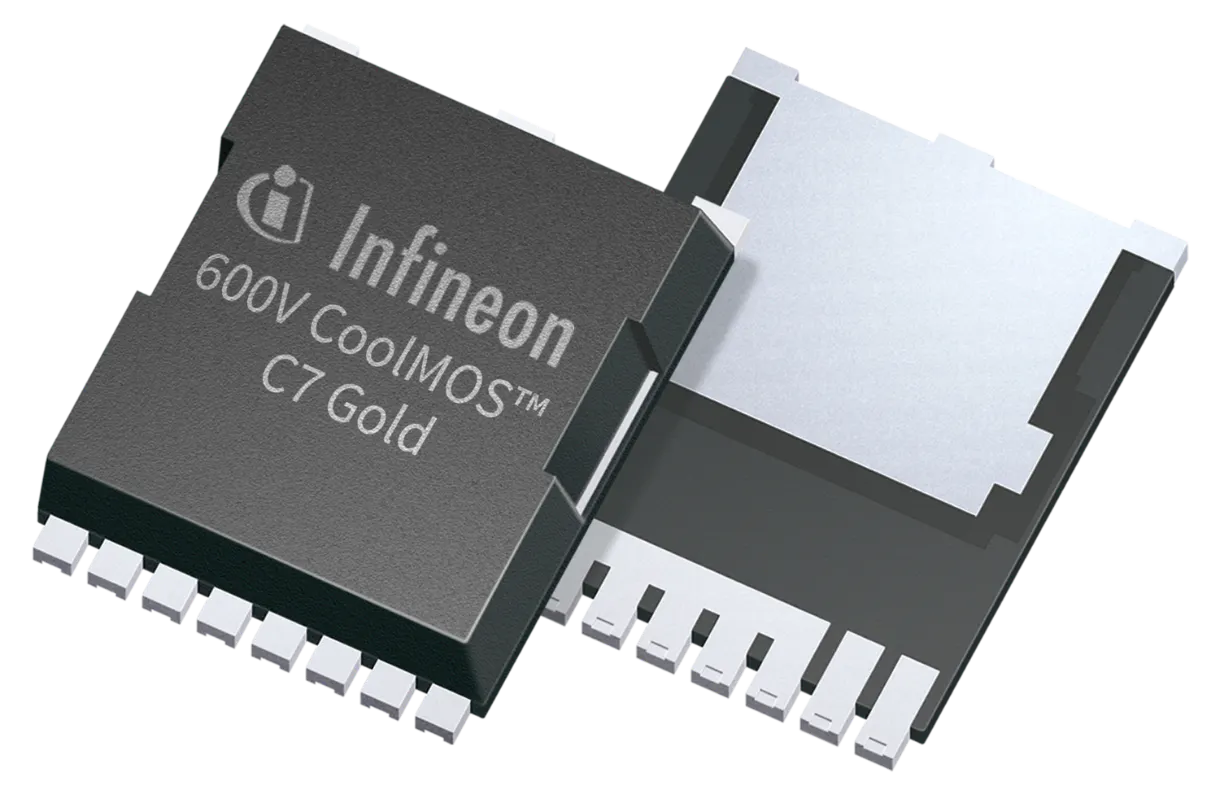Infineon CoolMOS C7 Gold SJ MOSFET
×1
 Resistor 4.7 ohm
×1

### Software apps and online servicesArduino IDE

### Hand tools and fabrication machinesSoldering iron (generic)

## Schematics

### Diagram for 12V Car Battery Solar Charge Controller

Diagram for build.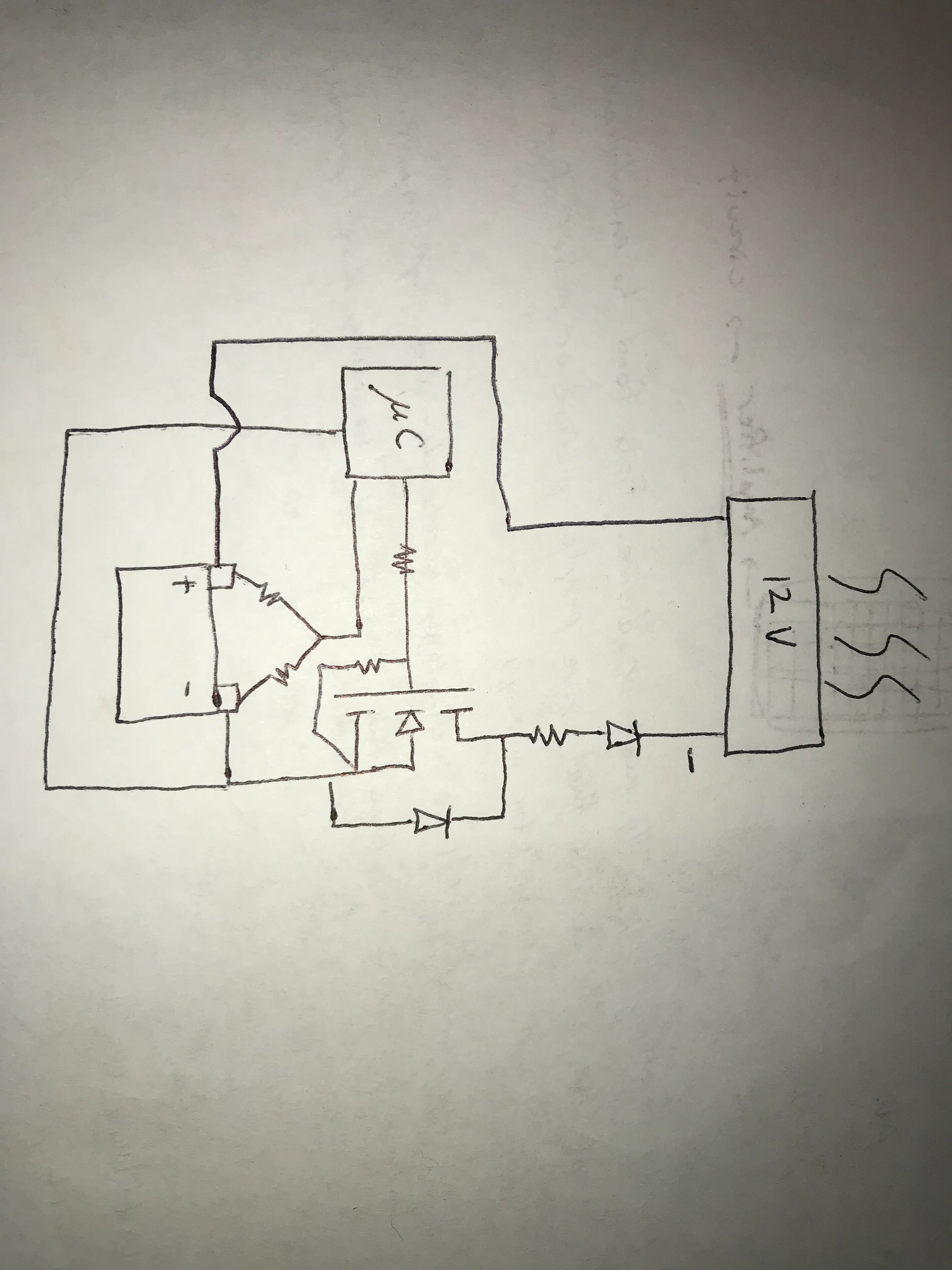## Code

### Code for 12V Car Battery Solar Charge Controller using Infineon MOSFET

C/C++
Use with Arduino IDE
```#define VOLTAGE A1 //voltage divider
#define MOSFET 2 //mosfet pin
float measure=0.0;  //value for battery voltage
float overVolt=14.0;  //overvoltage value
float underVolt=12.6; //undervoltage value
bool solarOn=LOW; //solar charging state so that charging can continue from undervolt to overvolt
bool ledOn=LOW;   //built-in LED state to enable blinking until overvolt
unsigned long int time1=0; //initial time
unsigned long int time2=0; //current time
unsigned long int interval=500;  //blink delay
void setup(){
pinMode(VOLTAGE,INPUT); //setup for voltage divider input
pinMode(MOSFET,OUTPUT); //setup mosfet for output
}

void loop(){
if(measure>overVolt){   //turn off charging if overvolt
solarOn=LOW;      //set solar state to off
ledOn=LOW;        //turn off built-in LED
}
if(measure<underVolt){    //turn on if voltage drops below undervolt
solarOn=HIGH;       //set solar state to on
}
if(solarOn==HIGH){     //separate condition from undervolt b/c you want built-in LED blinking until overvolt
time2=millis();     //get current time
if((time2-time1)>interval){   //if current time minus initial time is greater than interval
time1=time2;              //reset intial time to current time
ledOn=!ledOn;           //turn on or off led state
}
}
digitalWrite(MOSFET,solarOn);   //turn on or off mosfet
digitalWrite(LED_BUILTIN,ledOn); //turn on or off built-in LED
}
```

## Credits

### Edmund Celis

1 project • 1 follower
Please see my renewable energy vision. orionsarc.org